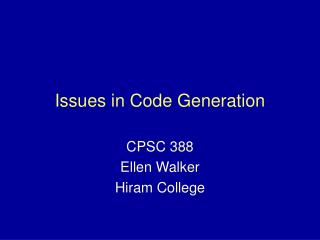# Issues in Code Generation - PowerPoint PPT PresentationDownload PresentationIssues in Code Generation

Issues in Code GenerationDownload Presentation## Issues in Code Generation

- - - - - - - - - - - - - - - - - - - - - - - - - - - E N D - - - - - - - - - - - - - - - - - - - - - - - - - - -
##### Presentation Transcript

1. Issues in Code Generation CPSC 388 Ellen Walker Hiram College

2. Address Calculation • Variables • Look up the “address” in the symbol table • Arrays & Structures • Look up base address, compute and add offset • Pointers • Generate code for indirect reference

3. Address Calculation Tools in 3-Address Code • Operators from C • &x address of x • *x value contained in location x • Addressing Modes • None data as before (x) • Address address of data (&x) • Indirect pointer to data (*x)

4. 3-Address Example: x=A[i] Assume: int A[i], sizeof(int) = 2 Allow the operator t = a[x] (array ref op) t1 = i * 2 compute index t2 = &A + t1 compute array addr t3 = *t2 get the value x = t3 assign to x

5. Address Calculation Tools in PCode • IND x • Add x to top of stack, use result as an address of item to push onto the stack • IXA x • Calculate address as (top)*x+(top-1), push address onto stack

6. Pcode Example: x=A[i] Assume: int A[i], sizeof(int) = 2 LDA x load address of x (for later LDA A load address of A LOD i load value of i IXA 2 calculate (&A+2*i) IND 0 Load item at that address STO store it in x

7. Pcode Generation for Arrays • Add subscript ([]) operation to syntax tree (OpKind = subs) • Add a parameter (isAddr) to the code generation function: • false return the value of the expression • true return the address of the expression • You get C-style multi-D arrays for free (arrays of arrays…)

8. New Case in GenCode case ‘[]’: //Array index cout << “LDA “ << t->first->name; Gen_code(t->first->next, false); cout << “IXA sizeof(“ << t->first->name << “)”; if (!isAddr) cout << “IND 0” break;

9. Revised to allow Multi-dimensional Arrays case ‘[]’: //Array index //push address gen_code(t->first, true); //push index gen_code(t->first->next, false); cout << “IXA sizeof(“ << t->first->name << “)”; if (!isAddr) cout << “IND 0” break;

10. Write Pcode for ... • A[i+1] = 2*A[i] • x = A[y][z] • Assume: • double A • sizeof(double) =4 • sizeof (double *) = 1

11. Class/Struct References • Address of element is base + offset • Base is address of class • Offset is sum of sizes of all elements previous (offset of 1st item is 0) • Pointer can be treated as struct with base 0, offset = pointer value!

12. Address computation • Assume sizeof int=2, sizeof double=4 • class st{ int A1, double A2, double A3}; • St x; • Addresses: • &(x.A1) = &x • &(x.A2) = &x + 2 • &(x.A3) = &x + 6

13. Offset computation • Add function: field_offset(st,field) returns the integer offset of field from the beginning of st • Computing the address of x.j • t1 = &x + field_offset (x,j) (3 addr) • LDA x; LOD field_offset(x,j); IXA 1 (Pcode)

14. Example (pointer & class) class tN{ int val; class tN *left; class tN *right; }; tN *p; p = p->right;

15. Pcode (p = p->right) • LDA p //for storing result • LOD p //push p (an address) • IND fieldoffset(tN,right); //*(p + offset) • STN // store in p

16. Generating Control Code • All control statements can be constructed from IF and WHILE • Two additional instructions are needed • FJP jump if false conditional jump • UJP jump unconditional jump • Need to be able to generate and assign labels to statements

17. Code for IF • if <E> <S1> else <S2> • In pcode: • Code to compute and push E • FJP Label1 • Code to execute S1 • UJP Label 2 • Label1: code to execute S2 • Label2:

18. Code for While • while <E> <S1> • In pcode: • Label1: Code to compute and push E • FJP Label2 • Code to execute S1 • UJP Label 1 • Label2: